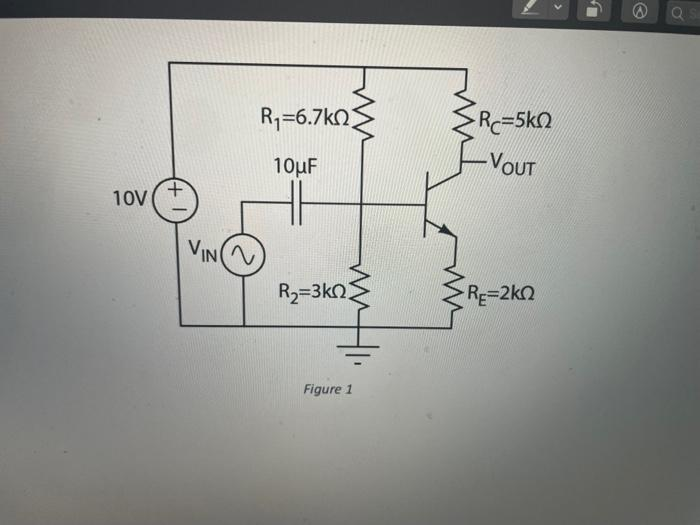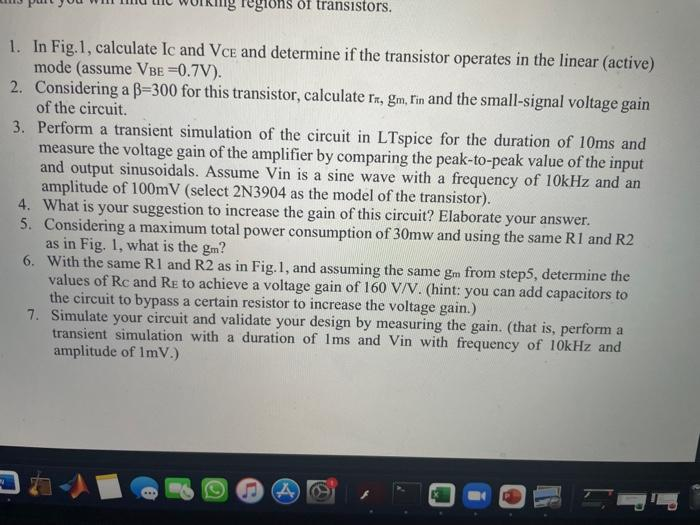# Question @ R=6.7kΩ Rc=5k02 10uF -VOUT 10V VINA R2=3k02 Re=2k02 Figure 1 Tegions of transistors. 1. In Fig. 1, calculate Ic and Vce and determine if the transistor operates in the linear (active) mode (assume VBE=0.7V). 2. Considering a B=300 for this transistor, calculate rx, gm, Iin and the small-signal voltage gain of the circuit. 3. Perform a transient simulation of the circuit in LTspice for the duration of 10ms and measure the voltage gain of the amplifier by comparing the peak-to-peak value of the input and output sinusoidals. Assume Vin is a sine wave with a frequency of 10kHz and an amplitude of 100mV (select 2N3904 as the model of the transistor). 4. What is your suggestion to increase the gain of this circuit? Elaborate your answer. 5. Considering a maximum total power consumption of 30mw and using the same R1 and R2 as in Fig. 1, what is the gm? 6. With the same R1 and R2 as in Fig. 1, and assuming the same gm from step5, determine the values of Rc and Re to achieve a voltage gain of 160 V/V. (hint: you can add capacitors to the circuit to bypass a certain resistor to increase the voltage gain.) 7. Simulate your circuit and validate your design by measuring the gain. (that is, perform a transient simulation with a duration of Ims and Vin with frequency of 10kHz and amplitude of ImV.) 94 5UT5HTC The Asker · Electrical EngineeringTranscribed Image Text: @ R=6.7kΩ Rc=5k02 10uF -VOUT 10V VINA R2=3k02 Re=2k02 Figure 1 Tegions of transistors. 1. In Fig. 1, calculate Ic and Vce and determine if the transistor operates in the linear (active) mode (assume VBE=0.7V). 2. Considering a B=300 for this transistor, calculate rx, gm, Iin and the small-signal voltage gain of the circuit. 3. Perform a transient simulation of the circuit in LTspice for the duration of 10ms and measure the voltage gain of the amplifier by comparing the peak-to-peak value of the input and output sinusoidals. Assume Vin is a sine wave with a frequency of 10kHz and an amplitude of 100mV (select 2N3904 as the model of the transistor). 4. What is your suggestion to increase the gain of this circuit? Elaborate your answer. 5. Considering a maximum total power consumption of 30mw and using the same R1 and R2 as in Fig. 1, what is the gm? 6. With the same R1 and R2 as in Fig. 1, and assuming the same gm from step5, determine the values of Rc and Re to achieve a voltage gain of 160 V/V. (hint: you can add capacitors to the circuit to bypass a certain resistor to increase the voltage gain.) 7. Simulate your circuit and validate your design by measuring the gain. (that is, perform a transient simulation with a duration of Ims and Vin with frequency of 10kHz and amplitude of ImV.) 94 5
More
Transcribed Image Text: @ R=6.7kΩ Rc=5k02 10uF -VOUT 10V VINA R2=3k02 Re=2k02 Figure 1 Tegions of transistors. 1. In Fig. 1, calculate Ic and Vce and determine if the transistor operates in the linear (active) mode (assume VBE=0.7V). 2. Considering a B=300 for this transistor, calculate rx, gm, Iin and the small-signal voltage gain of the circuit. 3. Perform a transient simulation of the circuit in LTspice for the duration of 10ms and measure the voltage gain of the amplifier by comparing the peak-to-peak value of the input and output sinusoidals. Assume Vin is a sine wave with a frequency of 10kHz and an amplitude of 100mV (select 2N3904 as the model of the transistor). 4. What is your suggestion to increase the gain of this circuit? Elaborate your answer. 5. Considering a maximum total power consumption of 30mw and using the same R1 and R2 as in Fig. 1, what is the gm? 6. With the same R1 and R2 as in Fig. 1, and assuming the same gm from step5, determine the values of Rc and Re to achieve a voltage gain of 160 V/V. (hint: you can add capacitors to the circuit to bypass a certain resistor to increase the voltage gain.) 7. Simulate your circuit and validate your design by measuring the gain. (that is, perform a transient simulation with a duration of Ims and Vin with frequency of 10kHz and amplitude of ImV.) 94 5